# 基于深度学习的大规模天线阵列混合波束赋形设计

### 文章目录

Code Guithub(记得关注师兄github以及本CSDN账户，博主更新不易，需要支持！): https://github.com/TianLin0509/BF-design-with-DL

# 系统模型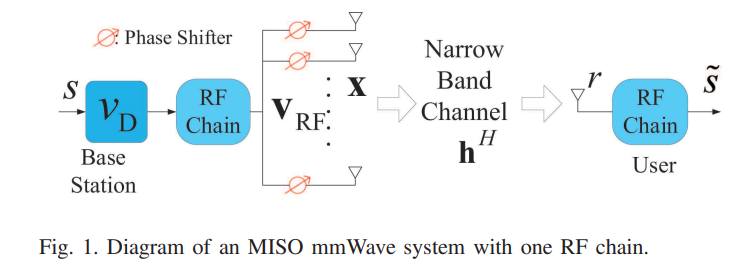$\mathbf{v}_{\mathrm{RF}}$：维度为$N_{\mathrm{t}} \times 1$的模拟预编码
$v_D$： 数字预编码权值（标量
$\mathbf{h}$ ：维度为$1 \times N_{\mathrm{t}}$ 的信道矩阵

$R=\log _{2}\left(1+\frac{1}{\sigma^{2}}\left\|\mathbf{h}^{H} \mathbf{v}_{\mathrm{RF}} v_{\mathrm{D}}\right\|^{2}\right)$

\begin{aligned} &\underset{\mathbf{v}_{\mathbf{R F}}}{\operatorname{maximize}} \quad \log _{2}\left(1+\frac{\gamma}{N_{t}}\left\|\mathbf{h}^{H} \mathbf{v}_{\mathbf{R F}}\right\|^{2}\right)\\ &\text { subject to } \quad\left|\left[\mathbf{v}_{\mathrm{RF}}\right]_{i}\right|^{2}=1, \quad \text { for } i=1, \ldots, N_{\mathrm{t}} \end{aligned}

# 文章贡献

• 新的设计方法：利用估计的CSI作为BFNN输入，直接输出最优beamforming权值
• 新颖的Loss函数：创新性地提出了与SE十分相关的一个Loss函数
• 对于非理性CSI的鲁棒性：提出了一种两阶段设计方法，利用估计的CSI作为输入，让BFNN学会接近理想CSI下的SE。在线部署阶段，BFNN能够适应非理性CS实现对信道估计误差的鲁棒性。

# NN（Neural Network）设计

## 一些挑战

BFNN的设计存在以下几个挑战：

1. BFNN的输入应该是什么？大多基于深度学习的工作是将接收到的基带信号作为输入，而接收信号本身也是$\mathbf{v}_{\mathrm{RF}}$的函数需要去优化。同时接收信号维度太低。
2. 如何保证BFNN的输出$\mathbf{v}_{\mathrm{RF}}$满足约束？大多深度学习（Deep Learning）如，tensoflow、pytorch框架并不支持复数输出。因此对输出施加恒模约束很困难。
3. 训练BFNN的时候如何打标签？大多传统的智能通信系统设计，标签是设定为传输比特或理想CSI。对于BF设计很难找到一个合适的标签（首先，目前到这篇博文为止无世界上最优的HBF，只有更优的HBF设计…其次，如果采用的传统HBF算法的$\mathbf{v}_{\mathrm{RF}}$为标签，无论怎么设计也不可能优于传统算法）

1. BFNN的输入：估计的信道矩阵$\mathbf{h}_{\mathrm{est}}$,估计的信噪比$\gamma_{\text {est }}$作为输入。
2. Lamda层满足恒模约束：经典的、完美的欧拉公式$\mathbf{v}_{\mathrm{RF}}=\exp (\mathrm{j} \cdot \boldsymbol{\theta})=\cos (\boldsymbol{\theta})+\mathbf{j} \cdot \sin (\boldsymbol{\theta})$将相位$\boldsymbol{\theta}$作为在最后一个Dense层的输出，然后添加一个基于欧拉公式的Lamda层满足恒模约束。（虽然理论公式简单…但是这种想法太妙，使得输出自然满足恒模约束！tql）
3. Loss 函数：在作者的设计中不需要标签，直接将Loss函数定义为：
$\text { Loss }=-\frac{1}{N} \sum_{n=1}^{N} \log _{2}\left(1+\frac{\gamma_{n}}{N_{\mathrm{t}}}\left\|\mathbf{h}_{n}^{H} \mathbf{v}_{\mathrm{RF}, n}\right\|^{2}\right)$
其中$N$是训练样本总个数，$\gamma_{n}$$\mathbf{h}_{n}$$\mathbf{v}_{\mathrm{RF},n},$分别代表第$n$个样本的SNR、CSI和输出模拟波束赋形权值。（注意：Loss函数的减少正好对应着平均SE的增加！）

## 两阶段设计方法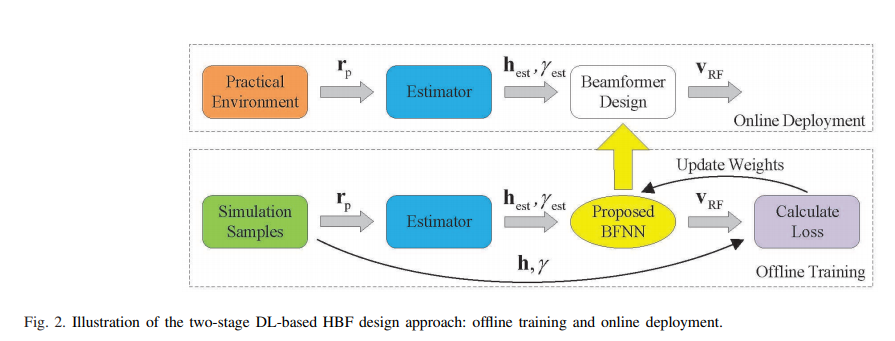1. 离线训练阶段：通过仿真，产生信道样本（理想CSI）、传输导频信号，噪声样本。然后在BS端实际的毫米波信道估计器利用获得部分的CSI（非理想CSI）。将估计的信道矩阵$\mathbf{h}_{\mathrm{est}}$,估计的信噪比$\gamma_{\text {est }}$作为BFNN的输入然后输出最优$\mathbf{v}_{\mathrm{RF},n},$最小化Loss函数。这里直接将理想CSI和SNR作为Loss的输入，这样做的好处是BFNN可以通过训练学会如何尽可能接近基于理想CSI下的SE从而对信道估计误差产生鲁棒性！
2. 在线部署阶段：实际的在线部署，BFNN的所有参数已被训练完毕并且固定，同时接收非理想CSI作为输入，直接输出模拟波束赋形权值。

# 仿真性能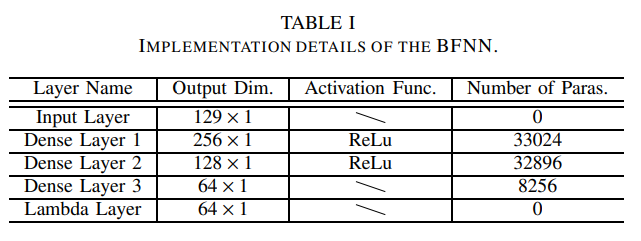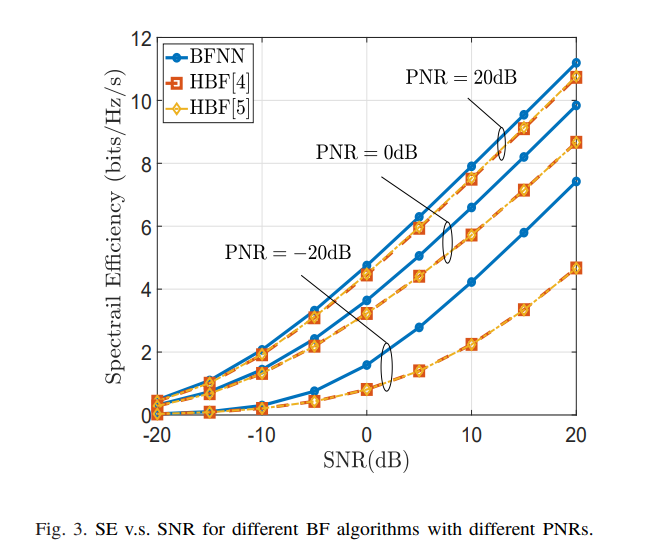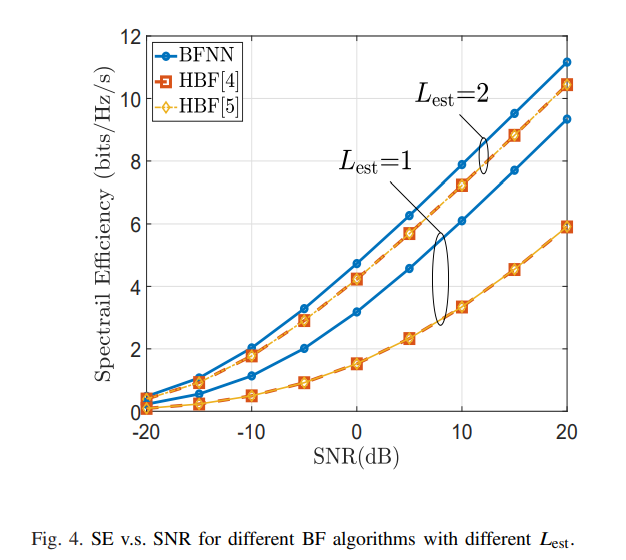# 结论

04-25676

#### 大规模天线阵列的原理、挑战和实现【转自微信公众号微波射频网】11-141766

#### 5G NR 学习记录（波束赋形）

05-023万+

#### 混合波束赋形专栏|基于坐标迭代更新法的混合波束赋形算法

04-21982

#### 无线系列 - 5G大规模MIMO阵列仿真及混合波束成形设计

02-273313

#### 基于深度学习的医学图像处理

06-132万+

#### 5G/NR 学习笔记: 波束赋形 / beam 管理

05-311043

#### 5G怎样实现波束赋形？©️2020 CSDN 皮肤主题: 大白 设计师: CSDN官方博客点击重新获取扫码支付1.余额是钱包充值的虚拟货币，按照1:1的比例进行支付金额的抵扣。
2.余额无法直接购买下载，可以购买VIP、C币套餐、付费专栏及课程。余额充值